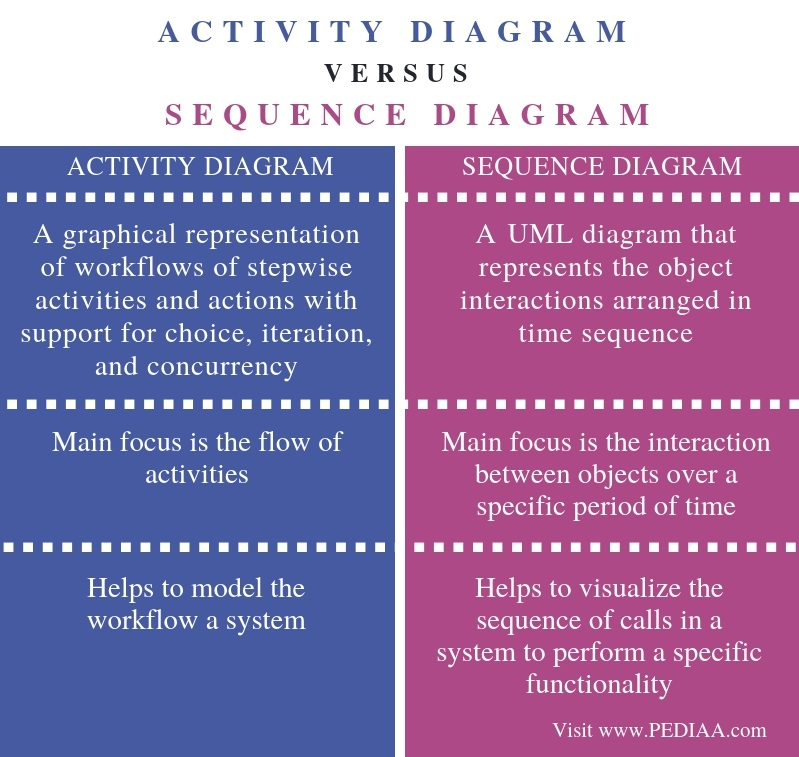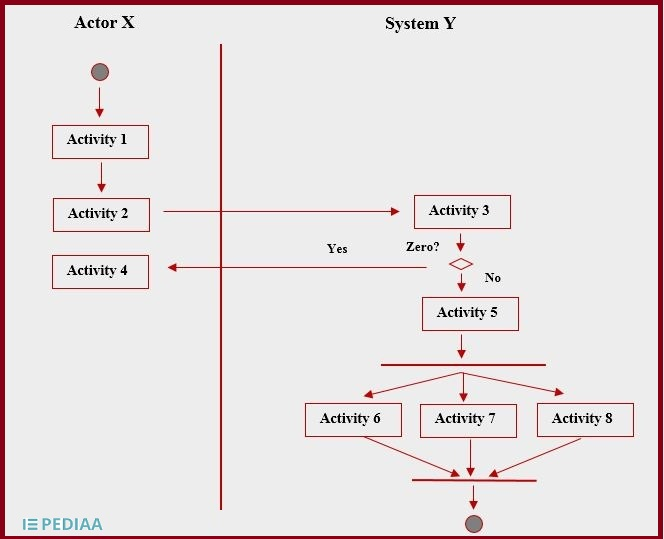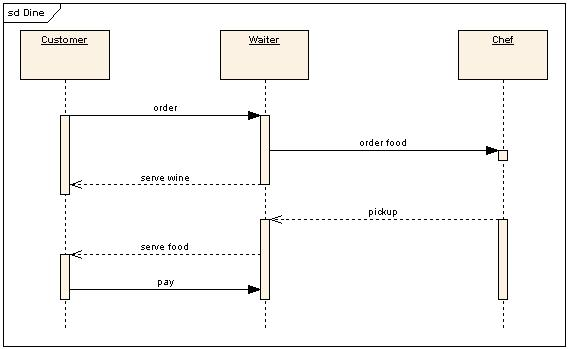# What is the Difference Between Activity Diagram and Sequence Diagram

The main difference between activity diagram and sequence diagram is that the activity diagram represents the flow of activities one after the other in a system while the sequence diagram represents the sequence of messages flowing from one object to another.

UML (Unified Modeling Language) is a standard language to visualize, construct and document software. It allows implementing object-oriented programming concepts to model the system. Moreover, it is easier to learn, and it provides a pictorial representation of the software. Furthermore, there are two types of UML diagrams; they are the structural and behavioral diagrams. Structural diagrams represent the static aspects of the system while behavior diagrams represent the dynamic aspects of the system. Activity and sequence diagrams are two behavior diagrams.

### Key Areas Covered

1. What is an Activity Diagram
– Definition, Functionality
2. What is a Sequence Diagram
– Definition, Functionality
3. What is the Difference Between Activity Diagram and Sequence Diagram
– Comparison of Key Differences

### Key Terms

Activity Diagram, Sequence Diagram, UML## What is an Activity Diagram

An activity diagram is a behavioral diagram that represents the flow of activities from one to another. Activities represent operations of the system. An example activity diagram is as follows.In the above diagram, the starting point is the initial node, while the arrow represents the flow of the activities. The diamond symbol represents a condition. If the condition is true, a set of activities will occur. If the condition is false, another set of activities will occur. After activity 5, there is a fork symbol. It is a division of activities. Then the join symbol combines multiple actions (activity 6, 7, 8) into one. Finally, the end symbol indicates the completion of an activity diagram.

## What is a Sequence Diagram

A sequence diagram is a behavior diagram that represents the interaction between objects over a specific period of time. In other words, it represents the sequence of messages flowing from one object to another.In the above sequence diagram, the arrows represent the request messages. The dashed arrows represent the return messages while the rectanglular vertical boxes denote the activation times of objects. The horizontal rectangle with the underlined text denotes the objects. When considering implementation and execution, it is important to discover the interactions between the components using a sequence diagram.

## Difference Between Activity Diagram and Sequence Diagram

### Definition

An activity diagram is a graphical representation of workflows of stepwise activities and actions with support for choice, iteration, and concurrency. On the other hand, a sequence diagram is a UML diagram that represents the object interactions arranged in time sequence. Thus, this is the main difference between activity diagram and sequence diagram.

### Main Focus

The main focus in an activity diagram is the flow of activities whereas the main focus in a sequence diagram is the interaction between objects over a specific period of time. Hence, this is also a major difference between activity diagram and sequence diagram.

### Usage

Another difference between activity diagram and sequence diagram is that an activity diagram helps to model the workflow a system while a sequence diagram helps to visualize the sequence of calls in a system to perform a specific functionality.

### Conclusion

Activity and sequence diagrams are two behavior diagrams. The main difference between activity diagram and sequence diagram is that the activity diagram represents the flow of activities in a system while the sequence diagram represents the sequence of messages flowing from one object to another.

##### Reference:

1. “Activity Diagram.” Wikipedia, Wikimedia Foundation, 14 Nov. 2018, Available here.
2. “Sequence Diagram.” Wikipedia, Wikimedia Foundation, 1 June 2018, Available here.

##### Image Courtesy:

1. “UML Sequence diagram” (CC BY-SA 3.0) via Commons Wikimedia### About the Author: Lithmee

Lithmee holds a Bachelor of Science degree in Computer Systems Engineering and is reading for her Master’s degree in Computer Science. She is passionate about sharing her knowldge in the areas of programming, data science, and computer systems.